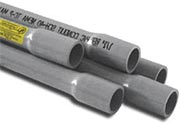# How to Calculate Conduit Fill

How-toCalculating conduit fill is a straightforward process, but important to comply with NEC requirements (National Electrical Code) and avoid expensive rewiring (and most importantly, to keep your electrical installation safe!). The following steps describe these factors and explains how to correctly calculate conduit fill.

There are three different factors that must be considered when performing raceway fill calculations:

1. Number of cables in the conduit
2. Cross section area of the cables
3. Number of bends in the conduit

## Step 1: Calculating wire cross-section area

If you already know how many wires you need (this means you know the number of wires and what insulation type and gauge these will be) all you need to do is find the cross-sectional area for each wire and add these up.

Example:

You have the following wire types and amounts:

• 4 wires, with insulation type THHN, and gauge of 8 AWG
• 2 wires, with insulation type THW, and gauge of 4 AWG

A 8AWG THHN wire has a cross section of 23.61 square millimeters (0.03659 square inches), while a 4 AWG THW has a cross section of 62.77 square millimeters (0.09729 square inches). This means the total cross section area of all the wires is:

(23.61 sq. mm) x 4      +   (62.77 sq. mm) x 2     =     219.98 sq. mm

## Finding minimum available conduit area

The National Electrical Code establishes that if a conduit only contains 1 wire, the max fill percentage is 53%. If it contains 2 wires, the max fill percentage is 31%. And if it contains 3 or more wires, the max fill percentage is 40%. Using the wire cross section areas calculated previously we can obtain the minimum conduit size we must use.

Example:

Following the previous example we noted that we’re using a total of 6 wires, which means that in our case, the max fill percentage is 40%. Since we had already calculated the total wire area, we can easily calculate the minimum conduit area:

219.98 sq. mm    /    0.4      =   549.95 sq. mm

Using a simple lookup, we can find the closest conduit size.

Example: If we need to use an EMT conduit, we can find that the closest conduit size is a 1 inch conduit, which gives us a 39% conduit fill.

## Planning for conduit bends

Bends in conduit make wire pulling hard, and a high conduit fill percentage makes it even harder, which is why even more empty conduit cross-section area is recommended in these cases. For each 90 degree conduit bend it is recommended to subtract 15% from the total cross-section area. Also, you should avoid whenever possible more than 2, 90 degree bends in a single section of conduit.